# Channel Reach Routing

Hydrology Studio employs the Modified Att-Kin (Attenuation Kinematic) and the Muskingum-Cunge methodologies for channel reach routing.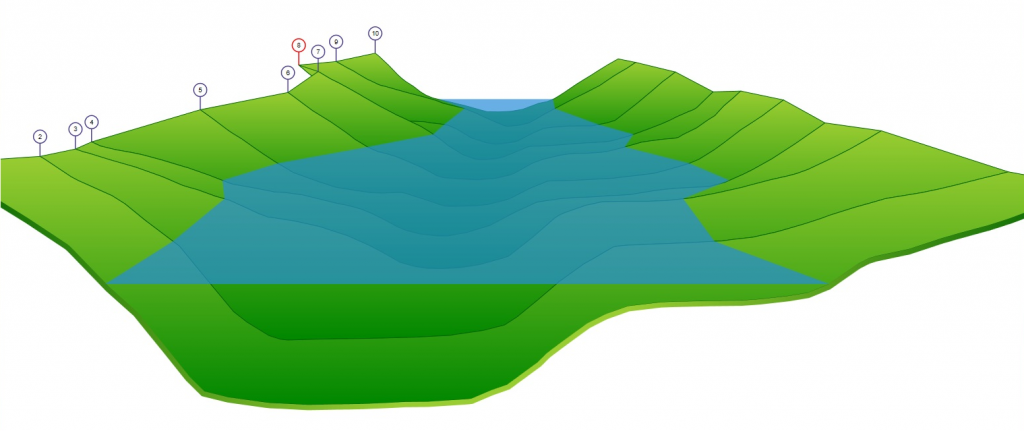Both theories are based on a discharge-flow area relationship where: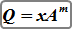The equations and formulas used to compute x and m are further described in Technical Release 20 (TR-20). Hydrology Studio allows you to optionally directly enter these variables when using the Modified Att-Kin method.

## Modified Att-Kin

The Modified Att-Kin method’s procedures are as follows: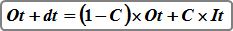Where:
Ot + dt = outflow at time t+dt
Ot = outflow at time t
It = inflow at time t
C = routing coefficient

The routing coefficient is computed as: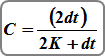Where:
dt = time interval in seconds or hours
K = L/(mV)

Where:
L = channel reach length (ft)
m = factor relating average velocity and wave velocity (celerity)
V = average velocity (ft/s)

## Muskingum-Cunge

The Muskingum-Cunge routing procedure has been incorporated into the NRCS TR20 hydrologic model. It will typically give higher peak flows than the Modified Att-Kin method. Both methods are more appropriate for modeling long channels.

Muskingum-Cunge computes outflows using four primary coefficients as shown in the following equation: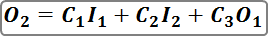Where:
O2 = Outflow discharge at time 2
O1 = Outflow discharge at time 1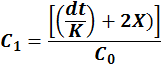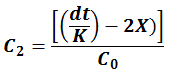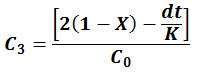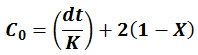Where:
dt = time interval in seconds
K & X are constants determined from: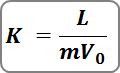Where:
L = length of the channel reach
m = factor relating average velocity and wave velocity (celerity)
V0 = average velocity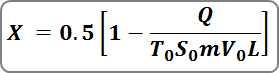Where:
Q = peak inflow
T0 = top width at Q
S0 = longitudinal slope